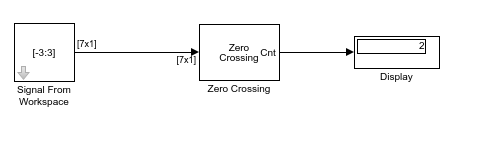# Detect Number of Zero Crossings in Signal

Use the Zero Crossing block to detect the number of times a signal crosses zero.

Open the model. Set the Stop Time of the model to 0 and run the model.

The Signal From Workspace block generates a 7-by-1 signal that goes from -3 to 3. This signal passes through zero once during the first time step. The Zero Crossing block detects this crossing and outputs the value in the Display block.To run the model for two time steps, change the simulation Stop Time to 1. The Zero Crossing block remembers that the last value of the last frame was 3. Therefore, the signal passes through zero twice during the second time step. It passes through zero while going from 3 to -3, and it passes through zero again while going from -3 to 3. The Zero Crossing block finds two zero crossings in the second time step as shown in the Display block in the following figure.